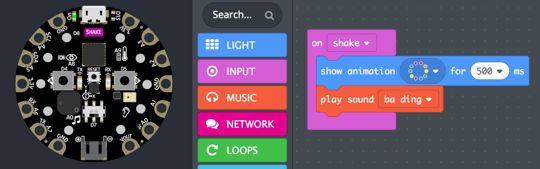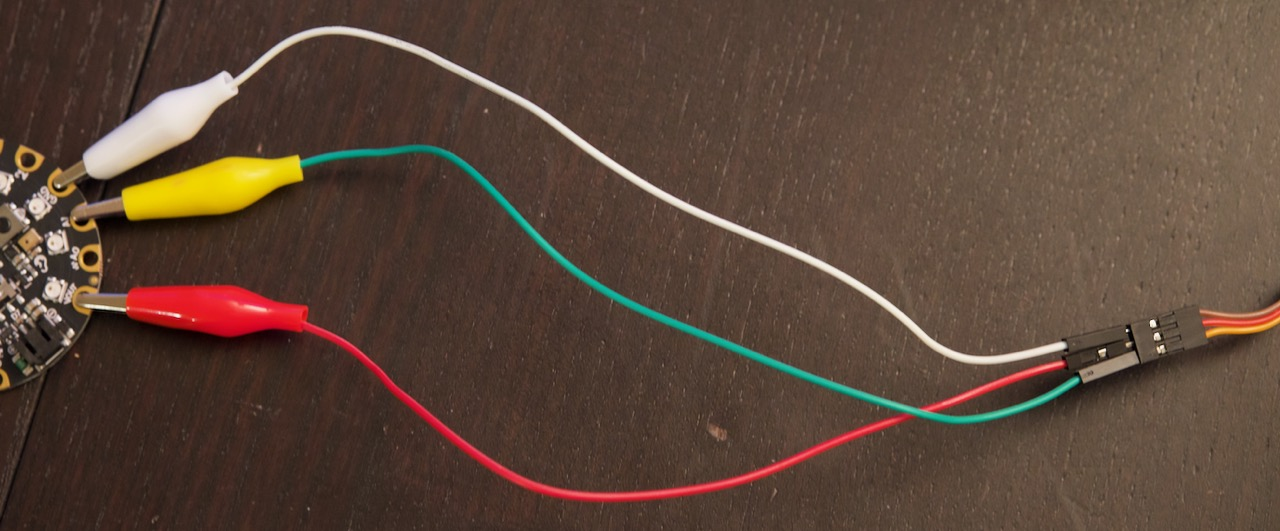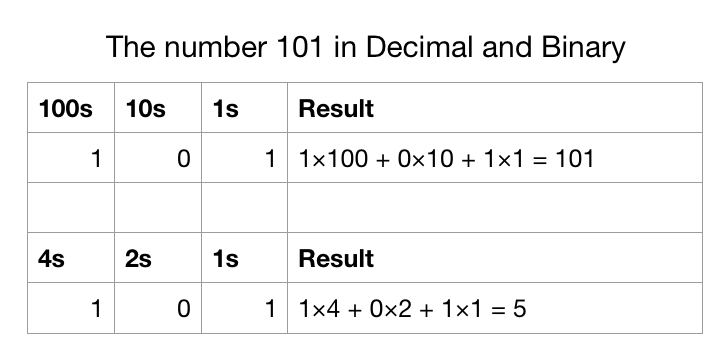↑ All Courses## Class Description

In this class, we will program the Circuit Playground Express, using blocks, JavaScript and Python. We’ll learn to use the accelerometer, microphone, speaker, buttons, switch, capacitive touch sensors, and temperature and light sensors with all sorts of fun projects.

## Oct 30

### More with Servo Motors

You can continue to explore using the Circuit Playground Express and a servo motor in two ways:

#### Using MakeCode

Here’s a sample to get you started.

Try using other inputs, such as light and sound to control the servo angle.

#### Using Python

This program has several operating modes (ways of working), and you can make your own. Copy and paste this code into code.py on the CPX. You only need to work with the lines below this one:
`# APPLICATION CODE STARTS HERE`

``````from random import randint, uniform, choice
import analogio, board, busio, digitalio, pulseio, adafruit_lis3dh
from time import sleep

MIN_PULSE, MAX_PULSE = 0.5, 2.4
PULSE_RANGE = MAX_PULSE - MIN_PULSE
FREQUENCY = 50
PERIOD_MS = 1.0 / FREQUENCY * 1000.0
SCALE = PERIOD_MS / 65535.0
PINS = (board.A1, board.A2, board.A3)

class Accel:
def __init__(self):
self._i2c = busio.I2C(board.ACCELEROMETER_SCL, board.ACCELEROMETER_SDA)
self._int1 = digitalio.DigitalInOut(board.ACCELEROMETER_INTERRUPT)

def value(self):
return self._lis3dh.acceleration

class Servo:
def __init__(self, pin = 1):
self.servo = pulseio.PWMOut(PINS[pin - 1], frequency=FREQUENCY)

def turn_to(self, angle):
pulse_ms = MIN_PULSE + (angle / 180) * PULSE_RANGE
self.servo.duty_cycle = int(pulse_ms / SCALE)

servo = Servo(1)
accel = Accel()

def map_values(i, i_min, i_max, o_min, o_max):
i_range = i_max - i_min
o_range = o_max - o_min
s = i - i_min
return min(o_max, max(o_min, o_min + s / i_range * o_range))

# APPLICATION CODE STARTS HERE

mode = 1  # Change this to try the different modes

while True:
if mode == 1:  # 0 to 180 by 26 (plays a neat rhythm)
for turn in range(0, 181, 26):
servo.turn_to(turn)
sleep(0.5 if turn == 0 else 0.2)

elif mode == 2:  # Turns randomly and sleeps randomly
servo.turn_to(uniform(0, 180))
sleep(uniform(0.1, 0.6))

elif mode == 3:  # Turns based on x acceleration
angle = map_values(accel.value().x, -9.81, 9.81, 0, 180)
servo.turn_to(angle)
sleep(0.05)
``````

## Oct 23

### Servo Motors and Animatronic Halloween Monsters

Next Tuesday after school we’re offering a class where we will make very simple animatronic Halloween monsters (inspired by an Adafruit lesson). Here you can see two of my simple (and not very scary) early creations.

Come to the class if you want to do the full project, but for today we’ll get some experience programming a servo motor.

#### Connecting the Motor to the Circuit Playground Express

With each Circuit Playground Express today there is a servo motor and and set of three wires, each with an alligator clip at one end. The color of the servo motor’s wires is important, but the color of the alligator clip wires is not.

Here’s how you connect things:

• Attach the alligator clips to VOUT, A1, and GND on the CPX
• Connect the other ends of the wires with alligator clips to the cable coming from the servo motor as follows:
• The brown servo wire goes to the wire connected to GND
• Red goes to VOUT
• Orange goes to A1#### Python Code to Control the Motor

If needed, review the instructions from last week so that you can edit code.py using TextEdit. Paste the following program into TextEdit and save it in code.py on the CIRCUITPY device:

``````import time, board, pulseio
from random import randint, uniform

MIN_PULSE, MAX_PULSE = 0.5, 2.4
PULSE_RANGE = MAX_PULSE - MIN_PULSE
FREQUENCY = 50
PERIOD_MS = 1.0 / FREQUENCY * 1000.0
SCALE = PERIOD_MS / 65535.0

servo = pulseio.PWMOut(board.A1, frequency=FREQUENCY)

def turn_to(angle):
pulse_ms = MIN_PULSE + (angle / 180) * PULSE_RANGE
servo.duty_cycle = int(pulse_ms / SCALE)

while True:
turn_to(randint(75, 105))
time.sleep(uniform(0.1, 0.6))
``````

Ignore everything above the comment “Your work goes here” for now. Let’s discuss the three lines below it.

Imagine that you are creating an animatronic Halloween monster like Mr. Briccetti’s, and that the servo motor is moving it. You might even sketch it.

• How would you like it to move?
• How should it turn?
• How long should it wait between turns?

Modify the code to make it work the way you want.

## Oct 16

### CircuitPython on the Circuit Playground Express

So far you’ve used MakeCode to program the CPX. Now we’ll learn how to use Python as well.

#### Installing CircuitPython

• Press reset on the CPX to get the green lights to appear
• The name of the device in the file system should change from `CPLAYBOOT` to `CIRCUITPY`

#### Make a Program

• Run the TextEdit application
• Use Spotlight (the magnifying glass icon at the top right) and start typing textedit until TextEdit appears
• Run TextEdit
• Choose Format -> Make Plain Text
• Paste in this program, replacing anything that is there:
``````from adafruit_circuitplayground.express import cpx
import time

cpx.pixels.brightness = 0.1
cpx.pixels = 255, 128, 0
time.sleep(60)
``````
• Save the program with Cmd-S, as code.py, on CIRCUITPY. It should run immediately and you should see that pixel 0 is now orange (made from 255 red, 128 green, and no blue)

#### Experiment with the Program

• Change the brightness (between 0 and 1)
• Turn on a different pixel (0 through 9)
• Turn on several pixels in different colors (0–255 each for red, green and blue)

## Oct 2

### Sending Infrared Messages

Continue your project to send a message with infrared light.

### Send Coded Message with Lights

Using a code where a is represented by decimal 1 or binary 1, b by 2 or binary 10, c by 3 or binary 11, and so on, display a message on your CPX. Here’s an example that shows “cab”:

You can modify it to show your own message.

#### Example: zoo

Let’s say you want to encode “zoo”. Zoo is the 26th letter of the alphabet. Convert 26 to binary. Consider the powers of two:

`16 8 4 2 1`

Find the largest of those that will divide into 26: 16.
26 - 16 = 10, so we have ten remaining

Find the largest power that will divide into 10: 8. 10 - 8 = 2

Find the largest power that will divide into 2: 2. 2 - 2 = 0

So 26, represented in binary, is

``````  16 8 4 2 1
1 1 0 1 0
``````

Or, `1 × 16 + 1 × 8 + 0 × 4 + 1 × 2 + 0 × 1`

## September 25

### Sending Infrared Messages

Let’s learn to use the blocks under Network. Write code to send and receive numbers. The numbers can mean anything. Here are some ideas, and you can make your own:

• Send a number that is a sound frequency. The receiver will play a tone at that frequency.
• Send a number that means how many times a light should blink
• Send a number that means which light should blink

## September 18

### Musical Instrument using the Accelerometer

Try it. Click on Edit, then read the instructions.

## September 11

### Representation Exercise

How can you represent something the CPX measures (light, sound, temperature, acceleration, etc.) with the NeoPixels (the ten LEDs) or the speaker? Different sounds for different ranges of values? Different brightness, colors, and number of lit NeoPixels?

Here is an example.

Modify my example or make something different.

## September 4

### A Secret Message with Five Lights

#### How Can We Represent the Whole Alphabet in Five Bits?

A bit is a binary digit: on or off, 1 or 0.

This CPX simulator has a secret message for you. The binary number representing the first letter in the message appears automatically. Press Button A to advance to the next letter, and Button B to restart at the beginning.

What is the message?## August 28

• Discussion: What did you enjoy and discover when programming the CPX last time? What problems did you have?
• Follow along and make: Tilting on the x axis turns on a pixel
• Challenges:
• Turn on pixels for tilting the other way on x, and on the y axis
• There are no pixels at the top and bottom positions, so you may want to light the two nearest ones
• Free exploration time

## August 21, 2019

• Introduction to the Circuit Playground Express
• Demo: Make a program, copy it to the CPX, share it and submit it
• Make something with blocks
• Test it in the simulator